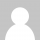# 函数式思维和函数式编程

``````quicksort :: Ord a => [a] -> [a]
quicksort [] = []
quicksort (p:xs) =
(quicksort lesser) ++ [p] ++ (quicksort greater)
where
lesser = filter (< p) xs
greater = filter (>= p) xs
``````

• 如果数组只有一个元素，返回这个数组
• 多于一个元素时，随机选择一个基点元素P，把数组分成两组。使得第一组中的元素全部 `<p`，第二组中的全部元素 `>p`。然后对这两组数据递归的使用这种算法。

``````public class Quicksort  {
private int[] numbers;
private int number;

public void sort(int[] values) {
if (values == null || values.length == 0){
return;
}
this.numbers = values;
number = values.length;
quicksort(0, number - 1);
}

private void quicksort(int low, int high) {
int i = low, j = high;
int pivot = numbers[low + (high-low)/2];

while (i <= j) {
while (numbers[i] < pivot) {
i++;
}
while (numbers[j] > pivot) {
j--;
}

if (i <= j) {
swap(i, j);
i++;
j--;
}
}
if (low < j)
quicksort(low, j);
if (i < high)
quicksort(i, high);
}

private void swap(int i, int j) {
int temp = numbers[i];
numbers[i] = numbers[j];
numbers[j] = temp;
}
}
``````

JAVA:

``````public class Quicksort {
private int[] numbers;

public void sort(int[] values) {

}
}
``````

``````quicksort :: Ord a => [a] -> [a]
``````

JAVA:

``````if (values.length == 0 || values == null) {
return;
}
``````

``````quicksort [] = []
``````

JAVA:

``````public void sort(int[] values) {
if (values.length == 0 || values == null) {
return;
}
this.numbers = values;
this.length = values.length;
quicksort(0, length - 1);
}
``````

``````No code. Nothing. Nada. That's good.
``````

JAVA:

``````private void quicksort(int low, int high) {
int i = low, j = high;
int pivot = numbers[low];

while (i <= j) {
while (numbers[i] < pivot) {
i++;
}
while (numbers[j] > pivot) {
j--;
}

if (i <= j) {
swap(i, j);
i++;
j--;
}
}

if (low < j)
quicksort(low, j);
if (i < high)
quicksort(i, high);
}
``````

``````private void swap(int i, int j) {
int temp = numbers[i];
numbers[i] = numbers[j];
numbers[j] = temp;
}
``````

``````quicksort (p:xs) = (quicksort lesser) ++ [p] ++ (quicksort greater)
``````

``````    where
lesser = filter (< p) xs
greater = filter (>= p) xs
``````

``````public List<Comparable> sort(List<Comparable> elements) {
if (elements.size() == 0) return elements;

Stream<Comparable> lesser = elements.stream()
.filter(x -> x.compareTo(pivot) < 0)
.collect(Collectors.toList());

Stream<Comparable> greater = elements.stream()
.filter(x -> x.compareTo(pivot) >= 0)
.collect(Collectors.toList());

List<Comparable> sorted = new ArrayList<Comparable>();

return sorted;

}
``````

 Haskell from scratch courtesy of “Learn you a Haskell for Great Good!”

 This quicksort in Haskell that I am showing here is not in-place quicksort so it loses one of its properties, which is memory efficiency. The in-place version in Haskell would be more like:

``````import qualified Data.Vector.Generic as V
import qualified Data.Vector.Generic.Mutable as M

qsort :: (V.Vector v a, Ord a) => v a -> v a
qsort = V.modify go where
go xs | M.length xs < 2 = return ()
| otherwise = do
p <- M.read xs (M.length xs `div` 2)
j <- M.unstablePartition (< p) xs
let (l, pr) = M.splitAt j xs
k <- M.unstablePartition (== p) pr
go l; go \$ M.drop k pr
``````

Discussion here.

 This version of quicksort is simplified for illustration purposes. It’s always good looking at the source. Boldly go and read this piece of History (with a capital H) by C.A.R. Hoare, “Quicksort”.

 Taken from http://www.vogella.com/tuto…..icksort/article.html

 Will we consider uncontrolled state harmful the same way `GOTO` statement being considered harmful consolidated structured programming?

 This wiki has LOTS of architectural information about the amazing Glasgow Haskell Compiler, `ghc`. https://ghc.haskell.org/trac/ghc/wiki/Commentary

 A big question mark over time on functional programming languages has been the ability (or lack thereof) to effectively code User Interfaces. Don’t despair though! There’s this cool new thing called Functional Reactive Programming (FRP). Still performing babysteps, but there are already implementations out there. One that’s gaining lots of momentum is ReactJS/Om/ClojureScript web app stack. Guess that might be a good follow-up post 🙂

### 13 Responses to 函数式思维和函数式编程

1.oscar says:

作为一个对Hashell语言彻头彻尾的新手

•Aqee says:

谢谢提醒，以更正

•amwyyyy says:

“已”写错了

•haha says:

哈哈， 你太QA了，哈哈

2.Yonghang Jiang says:

总觉得C++的话会用大堆的模板来实现它

3.ybsolar says:

```public void sort(int[] values) { if (values.length == 0 || values == null) { return; } this.numbers = values; this.length = values.length; quicksort(0, length - 1); }```这里的if判断有问题，如果values == null仍然会有空指针错误。

•says:

values == null要放前面

4. ss 对这篇文章的反应是不明觉厉
5.wk says:

前面的东西挑了那么多小错误，就没有人出来吐槽最后那段JAVA只是算法概要而不是真正的代码？

6. 桃园小七 对这篇文章的反应是好文
7. gason 对这篇文章的反应是赞一个
8.ccsdsx says:

public List sort(List list){
if(list == null || list.size() == 1){
return list;
}else{
Integer p = pivot(list);